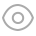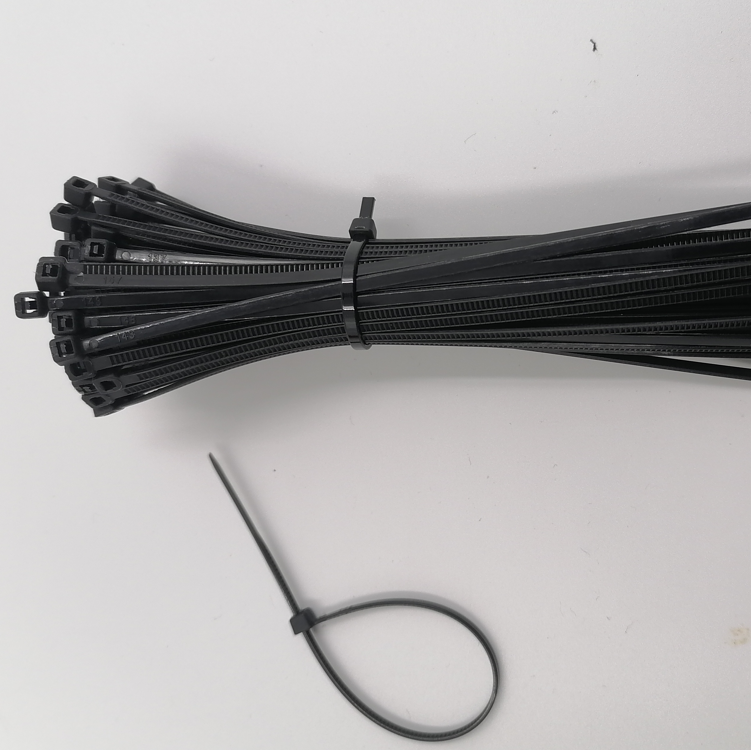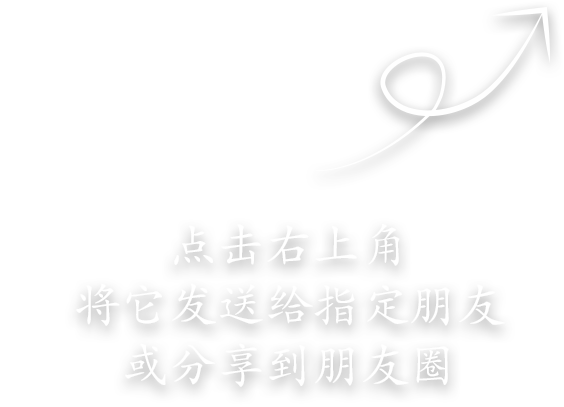• 手机版

用手机扫一扫

• 会员中心you postion

# Transformer transformation ratio

unknown unknown 发布日期：2023-01-30 21:48:1539

In general power transformers, the winding resistance voltage drop is very small and can be ignored, so the voltage U1=E1 can be considered in the primary winding. Because the secondary winding is open, the current I2=0, and its terminal voltage U2 is equal to the induced electromotive force E2, that is, U2=E2. Therefore, according to the above induced electromotive force formula of primary and secondary sides

Transformer transformation ratio

In the formula, K is the ratio of primary side voltage U1 and secondary side voltage U2, and the value of K is called the transformation ratio of transformer.

The above shows that the voltage ratio of the primary and secondary windings of the transformer is equal to the turn ratio of the primary and secondary windings, so if the primary and secondary windings have different voltages, just change their turns. When N1 ＞ N2, K ＞ 1, the transformer is depressurized; When N1 ＜ N2, K ＜ 1, the transformer is boosted.

For the supported transformer, its K is a constant value, so the secondary voltage is proportional to the primary voltage, that is, the secondary voltage increases and decreases with the increase of the primary voltage. However, the voltage at both ends of the loaded primary winding must be the rated value. Because when the applied voltage is slightly higher than the rated voltage, the current passing through the primary winding will increase greatly. If the transformer with the rated voltage of 220V is wrongly connected to the 380V line, the current of the primary winding will increase sharply, causing the transformer to burn out.K
thatis
n2

Shanghai Fakong Industrial and Trading Co.,Ltd.管理网站举报反馈网站统计